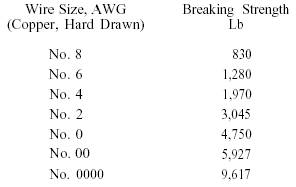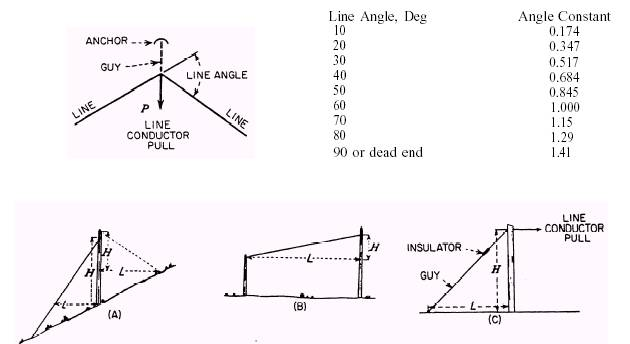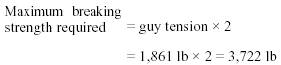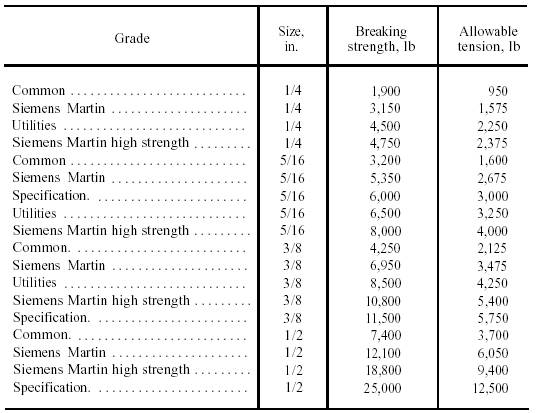Custom SearchTransformer InstallationConstruction Electrician BasicProtective DevicesTransformer Installation Rules

Some of the particularly important transformer installation rules are listed below.

1. One or more transformers may be hung on a single pole if the total weight does not exceed the safe strength of the pole or of the crossarms and bolts supporting them.

2. When more than one transformer is installed on crossarms, the weight should be distributed equally on the two sides of the pole.

3. Single-phase distribution transformers of 100 kVA or smaller are usually placed ABOVE the secondary mains if conditions permit. Those larger than 100 kVA are usually platform or pad mounted.

4. Lightning arresters and fused cutouts have to be installed on the primary side of all distribution transformers except the self-protected type.

5. Ground wires are required to be covered with plastic or wood molding to a point 8 feet above the base of the pole.

GUYING OF POLES

A guy is a brace or cable that is anchored in some fashion to the ground and secured to a point on the pole at the other end. Correctly selected and installed, the guy will protect the pole line from damage caused by the strain of the line conductors and pole-mounted equipment. The guy will also minimize the damage to the pole line caused by severe weather.

Basic guying information, such as types, locations, and anchors, is covered in Construction Electrician training manuals of lower rates. In this section, we'll be concerned with calculating the "line conductor load" for various line angles and dead ends, the effects that the lead-to-height ratios have on guy tensioning, and methods used to select the size and type of guy wire and anchors correctly.

The first step in determining the guy type and tension requirement is to determine the line conductor tension. Table 5-5 lists the most

Table 5-5.\Breaking Strength of Line Conductorscommon size of line conductors (hard-drawn copper) that you will encounter in the field. To determine the conductor tension under maximum loading conditions, take 50 percent or one-half of the breaking strength of the conductor. This allows for the safety factor of two required by the National Electric Safety Code.

Example:

Line tension = 50 percent of breaking strength

For No. 6 copper = 50 percent of 1,280 = 640 lb

Next, we must determine the angle of change in the line. Any change in the direction of the line increases the line conductor tension and, left uncorrected, tends to pull the pole out of alignment. Table 5-6 lists the most common line angles in degrees and the constant by which the line tension must be multiplied to determine the side pull.

Example: For No. 6 copper conductor, for a 30-degree angle,

640 0.517 = 330 lb

The total side pull can now be determined by multiplying the side pull of one conductor by the number of conductors.

Example: On the basis of four conductors, the total side pull is as follows:

330 4 = 1,320 lb

The next step required to determine the correct guy tension is to find the multiplying factor for the lead-to-height ratio. The lead-to-height ratio is the relationship of the lead (L) (distance between the base of the pole and the anchor rod) to the height (H) of the guy attachment on the pole, as shown in figure 5-16. This ratio will vary because the terrain of obstructions will restrict the location of the anchor. A guy ratio of 1 to 1 is preferred. Shortening L increases the tension in the guy, causing increased stresses on the pole especially at dead ends and acute angles.

Using our previous example of four No. 6 AWG copper conductors at a 30-degree angle, let's determine the total guy tension using a 1-to-1 ratio, assuming that H = 30 feet and L = 30 feet (refer to table 5-7). Locate the height of the guy attachment (30 ft) in the left-hand column. Move

Table 5-6.\Angle Constant Based on Line AngleFigure 5-16.\Methods of measuring height and lead dimensions.

Table 5-7.\Height and Distance Ratio Multiplierto the right until you reach the column under 30, the number of feet the anchor is away from the pole. The figure shown (1.41) is the guy ratio multiplier. Now let's compute the guy tensioning value.

Example: Total side pull guy ratio multiplier

1,320 1.41 = 1,861 lb

The guy wire and anchor for this example must be rated to hold at least 1,861 foot-pounds of load.

Guy wire comes in various sizes and grades from 1/4 to 1/2 inch. Table 5-8 lists the grades and sizes in the left-hand column with the breaking and allowable tension strengths in the right columns. To determine the correct grade and size of guy wire, first multiply the calculated guy tension by the safety factor of 2. Continuing with our example, solve for maximum breaking strength.

Example:We now know the guy wire must have a breaking strength of at least 3,722 pounds. Referring again to table 5-8, locate the breaking strength column; then move down this column until a value that is at least 3,722 pounds is found. Our example requires a breaking strength of 3,722 pounds. Based on this value, a 3/8-inch common grade would be sufficient.

The final step needed to ensure a safe and adequate guy is the selection of a guy anchor of sufficient holding power. The holding power of an anchor depends upon the area of the anchor plate, the depth setting, and the type of soil. The greater each of these is, the greater the volume of earth required to hold it in place. Table 5-9 lists the most commonly used manufactured anchors. To use this chart, determine the type of soil and

Table 5-8.\Guy Wire Breaking Strengthtotal guy tensioning amount. Move down the correct holding strength column until a value of at least the required amount is found. In the example just given, the guy tension allowed for is 3,722 pounds. Using table 5-9, we see that either an 8-inch expansion or screw would provide adequate holding power. The type selected would be based on material available, cost, and ease of installation. By following this five-step process closely, you can quickly determine the correct guy requirements for any situation.Integrated Publishing, Inc. - A (SDVOSB) Service Disabled Veteran Owned Small Business